# WBJEE 2020 Maths Paper with Solutions

WBJEE 2020 Maths question paper along with answer key and solutions are provided on this page. Students will greatly benefit from the solutions given here as they have been prepared by subject experts who have gone forward to make them as detailed and clear as possible. Students can make use of the WBJEE question paper to practise and learn these solutions so that they can easily tackle all types of questions in the upcoming WBJEE exams. They can also download the PDF version of the question paper to practise offline.

### WBJEE 2020 - Maths

Question 1. Let φ (x) = f(x) + f (1–x) and f"(x) < in [0,1], then

1. a. φ is monotonic increasing in [0,1/2] and monotonic decreasing in [1/2,1]
2. b. φ is monotonic increasing in [1/2,1] and monotonic decreasing in [0, 1/2]
3. c. φ neither increasing nor decreasing in any subinterval of [0,1]
4. d. φ neither is increasing in [0,1]

Solution:

φ (x) = f(x) + f(1–x)

Differentiate w.r.t. x

φ’(x) = f’(x) – f’(1–x) .....(i)

f’(x) -f’(1 – x) ≥ 0 (for monotonic increasing)

f’(x) ≥ f’(1– x), x ≤ 1 – x (since f’(x) is decreasing)

therefore x ≤ 1–x

2x ≤ 1

x ≤ 1/2 is monotonic increasing in [0,1/2] and monotonic decreasing in [1/2,1]

Question 2. Let cos–1(y/b) = log (x/n)n . Then

(Here yz = d2y/dx2, y1 = dy/dx)

1. a. x2 y2 + xy1 + n2y = 0
2. b. xy2–xy1 + 2n2y = 0
3. c. x2yz + 3xy1 -n2y = 0
4. d. xyz + 5xy1 - 3y = 0

Solution:

Given that cos-1(y/b) = log (x/n)n

Differentiate w.r.t. x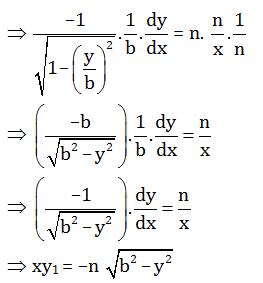Squaring both sides

x2y12 = n2 (b2–y2)

x2y12 = n2b2 – n2y2

Differentiate both sides

2xy12 +x2. 2y1. y2 = –n2. 2y. y1

xy1 + x2y2 = -n2y

x2y2 +xy1 + n2y = 0

Question 3.

$$\int \frac{f(x)\phi '(x)f'(x)}{(f(x)\phi (x)+1\sqrt{f(x)\phi (x)-1})}dx=$$

1. a. sin-1 √(f(x)/φ(x))+c
2. b. cos-1 √[(f(x))2-( φ(x)2 ]+c
3. c. √2 tan-1 √[(f(x)φ(x)-1)/2]+c
4. d. √2 tan-1 √[(f(x)φ(x)+1)/2]+c

Solution: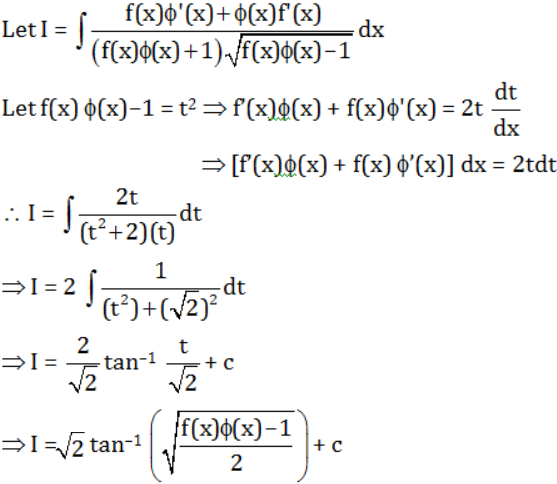Question 4. The value of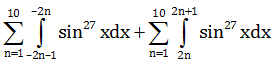is equal to:

1. a. 27
2. b. 54
3. c. –54
4. d. 0

Solution: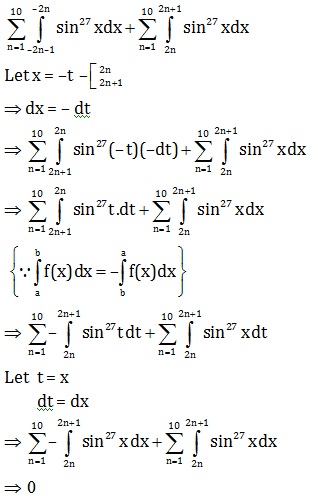Question 5.

$$\int_{0}^{2}[x^{2}]$$
is equal to:

1. a. 1
2. b. 5-√2- √3
3. c. 3-√2
4. d. 8/3

Solution: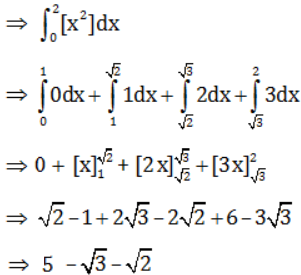Question 6. If the tangent to the curve y2=x3 at (m2, m3) is also a normal to the curve at (M2, M3), then the value of mM is :

1. a. -1/9
2. b. -2/9
3. c. -1/3
4. d. -4/9

Solution:

C: y2 = x3

Differentiate w.r.t. x

2y (dy/dx) = 3x2

(dy/dx) = 3x2/2y = m (slope)

Slope of tangent at (m2, m3)

⇒ m1 = 3(m2)2/2(m3)

⇒ m1 = 3m/2

Slope at (M2, M3)

⇒ m2 = 3(M2)2/2(M3)

⇒ m2 = 3M/2

Now tangent and normal are perpendicular to each other.

⇒ m1 m2 = –1

(3m/2)(3M/2) = –1

m M = -4/9

Question 7. If x2 + y2 = a2 then

$$\int_{0}^{a}\sqrt{1+(\frac{dy}{dx})^{2}dx}$$
=

1. a. 2πa
2. b. πa
3. c. πa/2
4. d. πa/4

Solution:

Given that

x2 + y2 = a2

Differentiate w.r.t. x

2x +2y (dy/dx) = 0

dy/dx = -x/y ..(1)

Now,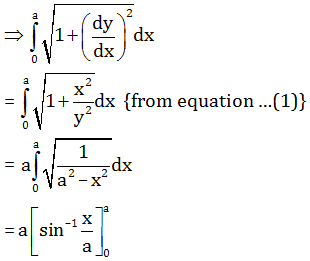= a(π/2)

= πa/2

Question 8. Let f, be a continuous function in [0,1] then

$$\lim_{n \to \infty }\sum_{i=0}^{n}\frac{1}{n}f(\frac{i}{n})$$

1. a.
$$\frac{1}{2}\int_{0}^{\frac{1}{2}}f(x)dx$$
2. b.
$$\int_{\frac{1}{2}}^{1}f(x)dx$$
3. c.
$$\int_{0}^{1}f(x)dx$$
4. d.
$$\int_{0}^{\frac{1}{2}}f(x)dx$$

Solution:

$$\lim_{n \to \infty }\sum_{i=0}^{n}\frac{1}{n}f(\frac{i}{n})$$

Let 1/n -> dx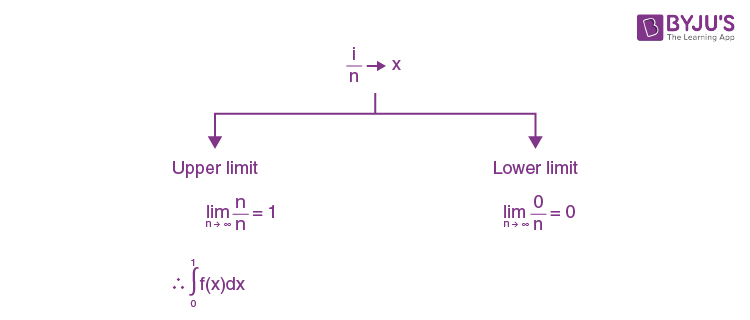Question 9. Let f be a differentiable function with

$$\lim_{x \to \infty }f(x)$$
= 0. If y'+ yf'(x) –f(x)f'(x) = 0,
$$\lim_{x \to \infty }y(x)=0$$
, then (where y' =dy/dx )

1. a. y+1 = ef(x) + f(x)
2. b. y–1 = ef(x) + f(x)
3. c. y+1 = e–f(x) + f(x)
4. d. y–1 = e–f(x) + f(x)

Solution:

y’ + y f’(x) – f(x)f’(x) = 0

(dy/dx) + yf’(x) = f(x)f’(x)

Compare to linear differential equation (dy/dx)+ py = q

So p = f’(x)

q = f(x) f’(x)

Integrating factor = e∫pdx = e∫f’(x)dx = ef(x)

Now, y.ef(x) = ∫ef(x).f(x).f’(x)dx

Let f(x) = t

f'(x) dx = dt

So y.ef(x) = ∫t.etdt+c

{Using by part}

yef(x) = tet-∫ etdt+c

yef(x) = et(t–1)+ c

yef(x) = ef(x) (f(x)–1) + c

{Given

$$\lim_{x \to \infty }f(x)=0$$
,
$$\lim_{x \to \infty }y(x)=0$$
}

⇒(0)(e0) = e0(0–1) + c

⇒ c = 1

∴ yef(x) = ef(x)(f(x)–1) +1

y = (f(x)–1) + e–f(x)

y + 1 = f(x) + e–f(x)

Question 10. Let f(x) = 1- √(x2) where the square root is to be taken positive, then

1. a. f has no extrema at x = 0
2. b. f has minima at x = 0
3. c. f has maxima at x = 0
4. d. f' exist at 0

Solution:

f(x) = 1- √(x)2

f(x) = 1- |x |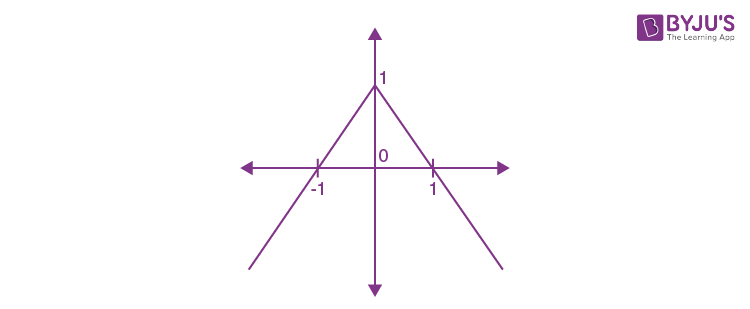Therefore f(x) is maximum at x = 0 and is 1.

Question 11. If x sin (y/x)dy = [y sin(y/x)-x] dx, x > 0 and y(1) = π/2 then the value of cos (y/x) is

1. a. 1
2. b. Log x
3. c. e
4. d. 0

Solution:

x sin(y/x)dy = [y sin (y/x)-x]dx

sin (y/x)dy/dx = (1/x)[y sin (y/x)-x]

sin (y/x)(dy/dx) = (y/x)sin (y/x)-1

dy/dx = [(y/x) sin(y/x)-1]/ sin(y/x)

Let y/x = v

⇒ dy/dx = v+x(dv/dx)

v+x(dv/dx) = (v sin v-1)/sin v

⇒ x dv/dx =[( v sin v-1)/sin v]-v

= -1/sin v

Integrate both sides

⇒ - ∫sin v dv = ∫(1/x)dx

⇒ cos v = log x + c

⇒ cos y/x = log x + c

{ given y(1)=π/2 ⇒ x = 1, y = π/2}

∴ cos π/2 = log 1 + c ⇒ c = 0

⇒ cos y/x = log x

Question 12. If the function f(x) = 2x3-9ax2+12a2x +1[a > 0] attains its maximum and minimum at p and q respectively such that p2 = q, then a is equal to

1. a. 2
2. b. 1/2
3. c. 1/4
4. d. 3

Solution:

f(x) = 2x3-9ax2+12a2x + 1

Differentiate w.r.t. x

f’(x) = 6x2–18ax+12a2 …….(1)

For extreme values

⇒ f’(x) = 0

6x2–18ax+12a2 = 0

⇒ x2–3ax+12a2 = 0

⇒ (x-a) (x-2a) = 0

⇒ x = a, 2a

Again differentiate equation (1) w.r.t. x

f”(x) = 12x-18 a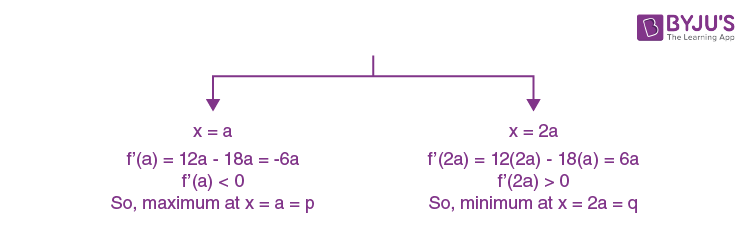Now,

⇒ P2 = q

⇒ (a)2 = 2a

⇒ a = 2

Question 13. If a and b are arbitrary positive real numbers, then the least possible value of (6a/5b)+(10b/3a) is:

1. a. 4
2. b. 6/5
3. c. 10/3
4. d. 68/15

Solution:

Using the concept of A.M ≥ GM

A.M ≥ GM

⇒ [(6a/5b)+(10b/3a)]/2 ≥ [(6a/5b)(10b/3a)]1/2

⇒ (6a/5b)+(10b/3a) ≥2(4)1/2

⇒ (6a/5b)+(10b/3a) ≥ 4

The least possible value of (6a/5b)+(10b/3a) is 4.

Question 14. If 2log(x+1)–log(x2–1)–log2, then x =

1. a. Only 3
2. b. –1 and 3
3. c. only -1
4. d. 1 and 3

Solution:

2 log (x+1)-log (x2-1) = log2

⇒log (x+1)2-log (x2-1) = log2

⇒log (x+1)2/(x2-1) = log2

⇒ (x+1)2/(x2-1) = 2

⇒ x+1 = 2x-2

⇒ x = 3

Question 15. The number of complex number p such that |p| = 1 and imaginary part of p4 is 0, is

1. a. 4
2. b. 2
3. c. 8
4. d. Infinitely many

Solution:

Let p = x+iy

Squaring both sides

p2 = x2+i2y2+2ixy

p2 = x2-y2+2ixy

Squaring both side

p4 = [(x2-y2) + 2ixy]2

p4 = (x2-y2)2 +(2ixy)2+4ixy(x2-y2)

Imaginary part of p4 is 0

xy(x2-y2) = 0

x3y-xy3 = 0

x = ±y

since |p| = 1

⇒ x2 + y2 = 1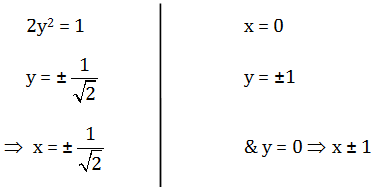Total value of p is 8.

Question 16. The equation

$$z\bar{z}(2-3i)z+(2+3i)\bar{z}+4=0$$
represents a circle of radius

1. a. 2 unit
2. b. 3 unit
3. c. 4 unit
4. d. 6 unit

Solution:

Given that

$$z\bar{z}(2-3i)z+(2+3i)\bar{z}+4=0$$

Since centre and radius of

$$z\bar{z}+\bar{a}z+a\bar{z}+b=0$$
are -a and
$$\sqrt{a\bar{a}-b}$$

$$\sqrt{(2-3i)(2+3i)-4}$$

=

$$\sqrt{13-4}$$

= √9

= 3 Unit

Question 17. The expression ax2+bx+c (a, b and c are real) has the same sign as that of a for all x is

1. a. b2-4ac > 0
2. b. b2-4ac ≠ 0
3. c. b2–4ac ≤ 0
4. d. b and c have the same sign as that of a

Solution:

Given that

ax2+bx+c has the same sign

Case-I: If a > 0, ax2+bx+c > 0, So b2-4ac < 0Case-II: If a < 0, ax2 +bx + c ≤ 0 So D ≤ 0Question 18. In a 12 storied building, 3 persons enter a lift cabin. It is known that they will leave the lift at different floor. In how many ways can they do so if the lift does not stop at the second floor?

1. a. 36
2. b. 120
3. c. 240
4. d. 720

Solution:

The lift can stop at 12–1–1 = 10 floors (except the floor they enter and the second floor)

Total number of ways = 10P3

= 10 × 9 × 8 = 720

Question 19. If the total number of m-element subsets of the set A = {a1, a2, ........., an} is k times the number of m element subsets containing a4 then n is

1. a. (m–1)k
2. b. mk
3. c. (m + 1)k
4. d. (m+2)k

Solution:

Set A = {a1, a2, a3, a4, ……. , an}

From set of n element selecting a subset of m element = nCm

Now, a4 is already selected.

So total number of sets which contains an is n–1Cm–1

Now, it is given that

nCm = K. n–1Cm–1

n!/m!(n-m)! = K(n-1)!/(m-1)!(n-m)!

n/m = k

n = mk

Question 20. Let I(n) = nn, J(n) = 1.3.5. ....(2n–1) for all (n > 1), n∈N, then

1. a. I(n) > J(n)
2. b. I(n) < J(n)
3. c. I(n) ≠ J(n)
4. d. I(n) = (½)J(n)

Solution:

Using the concept of A.M. ≥ G.M.

For J(n) A.m. > G. m.

⇒ (1+3+5+…+2n-1)/n > (1.3.5. ……(2n–1))1/n

⇒ n2/n > (J(n))1/n

⇒ n > (J(n))1/n

⇒ nn > J(n)

I(n) > J(n)

Question 21. If C0, C1, C2 ,.....,C15 are the Binomial co-efficients in the expansion of (1 + x)15, then the value of C1/C0 + 2C3/C1+3C3/C2 +….+15C15/C14 is

1. a. 1240
2. b. 120
3. c. 124
4. d. 140

Solution: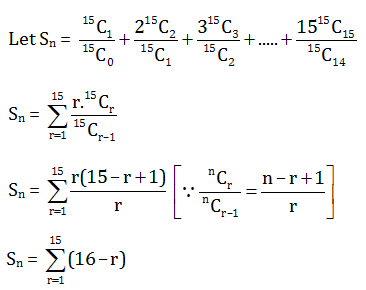Sn = 1 + 2 + 3 + 4 + …….. + 15

Sn = 15 x 16/2

Sn = 120

Question 22. Let A =

$$\begin{bmatrix} 3-t & 1 & 0\\ 1 & 3-t & 1\\ 0 & -1& 0 \end{bmatrix}$$
and det A = 5, then

1. a. t = 1
2. b. t = 2
3. c. t = –1
4. d. t = –2

Solution:

A =

$$\begin{bmatrix} 3-t & 1 & 0\\ 1 & 3-t & 1\\ 0 & -1& 0 \end{bmatrix}$$

|A| = –1 [(–1)(3–t)–0]

⇒ 5 = –1(t –3)

⇒ 5 = 3–t

⇒ t = –2

Question 23. Let A =

$$\begin{bmatrix} 12 &24 &5 \\ x& 6 & 2\\ -1&-2 & 3 \end{bmatrix}$$
. The value of x for which the matrix A is not invertible is

1. a. 6
2. b. 12
3. c. 3
4. d. 2

Solution:

A =

$$\begin{bmatrix} 12 &24 &5 \\ x& 6 & 2\\ -1&-2 & 3 \end{bmatrix}$$

if matrix is not invertible

⇒ |A| = 0

So |A| =

$$\begin{vmatrix} 12 & 24 & 5\\ x&6 & 2\\ -1& -2 & 3 \end{vmatrix}=0$$

⇒ [–1(48–30) +2(24–5x)+3(72-24x)] = 0

⇒ –18+48–10x+216-72 x = 0

–82x = –246

x = 3

Question 24. Let A =

$$\begin{bmatrix} a & b\\ c & d \end{bmatrix}$$
be a 2 × 2 real matrix with det A = 1. If the equation det (A–λI2) = 0 has imaginary roots (I2 be the identity matrix of order 2), then

1. a. (a + d)2 < 4
2. b. (a + d)2 = 4
3. c. (a + d)2 > 4
4. d. (a + d)2 = 16

Solution:

$$\begin{bmatrix} a & b\\ c & d \end{bmatrix}$$

Det A =

$$\begin{vmatrix} a &b \\ c& d \end{vmatrix}$$
= 1

det A = ad -cb = 1 …….(1)

Now,

A- λI2 = 0

$$\begin{bmatrix} a & b\\ c & d \end{bmatrix}-\lambda \begin{bmatrix} 1 &0 \\ 0 & 1 \end{bmatrix}=0$$

$$\begin{bmatrix} a-\lambda & b\\ c & d-\lambda \end{bmatrix}=0$$

$$\begin{vmatrix} a-\lambda&b \\ c& d-\lambda \end{vmatrix}=0$$

(a - λ) (d-λ)-cb = 0

λ2– λ(a + d) + ad – cb = 0

λ2– λ(a + d) + 1 = 0 {from eq. (1)}

Since roots are imaginary

D < 0

(a + d)2 -4(ad–cb)< 0

(a + d)2 < 4

Question 25. If

$$\begin{bmatrix} a^{2} & bc & c^{2}+ac\\ a^{2}+ab & b^{2} & ca\\ ab& b^{2}+bc & c^{2} \end{bmatrix}$$
= ka2b2c2, then k is equal to:

1. a. 2
2. b. –2
3. c. –4
4. d. 4

Solution: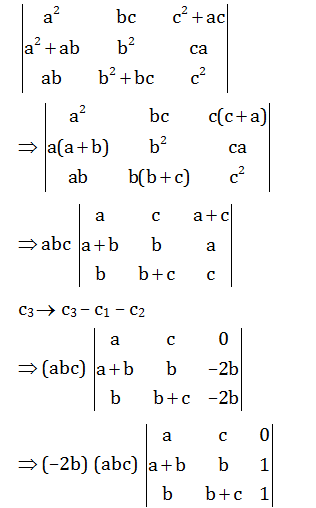= (–2b) (abc) (-2ac)

= 4a2b2c2

So, 4a2b2c2 = ka2b2c2

k = 4

Question 26. If f : S -> R where S is the set of all non-singular matrices of order 2 over R and

$$f\begin{bmatrix} a&b \\ c& d \end{bmatrix}$$
= ad – bc, then

1. a. f is a bijective mapping
2. b. f is one-one but not onto
3. c. f is onto but not one-one
4. d. f is neither one-one nor onto

Solution:

Given that

$$f\begin{bmatrix} a&b \\ c& d \end{bmatrix}$$

$$f\begin{bmatrix} 2&0 \\ 0& 2 \end{bmatrix}$$
= 4-0 = 4

$$f\begin{bmatrix} 4&0 \\ 0& 1 \end{bmatrix}$$
= 4-0 = 4

Hence not one-one function

As O ∈R but s does not contain any singular matrix so, f is not onto.

Question 27. Let the relation be defined on R by a ρ b holds if and only if a – b is zero or irrational then

1. a. ρ is an equivalence relation
2. b. ρ is reflexive & symmetric but is not transitive
3. c. ρ is reflexive and transitive but is not symmetric
4. d. ρ is reflexive only

Solution:

If a-b = 0 then b-a = 0

If a-b is irrational then b-a is irrational

Therefore, a ρ b -> b ρ a ⇒ symmetric

∀a ∈ R, a – a = 0, a ρ a ⇒ reflexive

If a = 2, b = √2 , c = 3 then

a ρ b, b ρ c but a ρ c is not true ⇒ not transitive.

Question 28. The unit vector in ZOX plane, making angles 450 and 600 respectively with

$$\vec{\alpha }= 2\hat{i}+2\hat{j}-\hat{k}$$
and
$$\vec{\beta }= \hat{j}-\hat{k}$$

1. a.
$$\frac{1}{\sqrt{2}}\hat{i}+\frac{1}{\sqrt{2}}\hat{j}$$
2. b.
$$\frac{1}{\sqrt{2}}\hat{i}-\frac{1}{\sqrt{2}}\hat{k}$$
3. c.
$$\frac{1}{\sqrt{2}}\hat{i}-\frac{1}{\sqrt{2}}\hat{j}$$
4. d.
$$\frac{1}{\sqrt{2}}\hat{i}+\frac{1}{\sqrt{2}}\hat{k}$$

Solution:

Given that

$$\vec{\alpha }= 2\hat{i}+2\hat{j}-\hat{k}$$

$$\left | \vec{\alpha } \right |= \sqrt{4+4+1}=3$$

$$\vec{\beta }= \hat{j}-\hat{k}$$

$$\left | \vec{\beta } \right |= \sqrt{1+1}= \sqrt{2}$$

Let the vector be

$$\left | \vec{\gamma } \right |= x\hat{i}+y\hat{j}$$

$$\left |\vec{\gamma } \right |= 1$$

{Vector in zox plane}

$$\vec{}\gamma .\vec{}\alpha =\left |\vec{\gamma } \right |\left |\vec\alpha \right |cos 45^{0}$$

$$(x\hat{i}+z\hat{k})(2\hat{i}+2\hat{j}-\hat{k})=3\times \frac{1}{\sqrt{2}}$$

2x-z = 3/√2 …(1)

$$\vec{\gamma }.\vec{\beta }=\left | \gamma \right |\left | \beta \right |\cos 60^{0}$$

$$(x\hat{i}+z\hat{k})(\hat{j}-\hat{k})=1(\sqrt{2})(\frac{1}{2})$$

z = -1/√2 …(2)

Comparing equation (1) and (2)

x = 1/√2

hence

$$\vec{\gamma }=\frac{1}{\sqrt{2}}\hat{i}-\frac{1}{\sqrt{2}}\hat{k}$$

Question 29. Four persons A, B, C and D throw an unbiased die, turn by turn, in succession till one get an even number and win the game. What is the probability that A wins if A begins?

1. a. 1/4
2. b. 1/2
3. c. 7/12
4. d. 8/15

Solution:

A wins 1st attempt P(even number) = 1/2

P(odd number) = 1– ½ = 1/2

P(A win) =

$$P(A)+P(\bar{A})P(B)P(\bar{C})P(D)P(A)+..$$

(A win ) = ½ +(1/2)(1/2)(1/2)(1/2)(1/2)+….

= ½ +(1/2)4(1/2) +(1/2)8(1/2)+…

= ½ +(1/2)5+(1/2)9+….

Using the concept of sum of infinite G.P.

P(A win) = a/1-r

= (½)/(1-(1/2))4

= (½)(16/15)

= 8/15

Question 30. The rifleman is firing at a distant target and has only 10% chance of hitting it. The least number of rounds he must fire to have more than 50% chance of hitting it at least once is

1. a. 5
2. b. 7
3. c. 9
4. d. 11

Solution:

P (hitting a target) = 1/10

∴P (not hitting a target) = 1 – 1/10 = 9/10

∴ Let number of trials = n

Now, P (missing all) + P (hitting at least once) = 1

P(hitting at least once) = 1 – P(missing)

= 1-(9/10)n ≥ 1/2

⇒ (0.9)n ≤ 0.5

Now, n = 6 ⇒ (0.9)6 = 0.531441

n = 7 ⇒ (0.9)7 = 0.4782969

∴ Require at least 7 shots

Question 31. cos(2x+7) = a (2–sinx) can have a real solution for

1. a. all real values of a
2. b. a ∈ [2, 6)
3. c. a ∈ [–∞, 2]\{0}
4. d. a ∈(0, ∞)

Solution:

Bonus

Question 32. The differential equation of the family of curves y = ex (A cos x + B sin x) where A, B are arbitrary constant is

1. a. (d2y/dx2 )-9x =13
2. b. (d2y/dx2 )-2dy/dx + 2y = 0
3. c. (d2y/dx2 )+ 3y = 4
4. d. (dy/dx)2+(dy/dx)– xy = 0

Solution:

y = ex(A cos x+B sin x)

Differentiate w.r.t.x

⇒ (dy/dx) = ex(A cos x+B sin x)+ex(-A sin x+B cos x)

⇒ dy/dx = y+ex(-A sin x+B cos x)

Again differentiate w.r.t.x

⇒ (d2y/dx2) = (dy/dx)+ex(-A sin x+B cos x)+ex(-Acos x-B sin x)

⇒ (d2y/dx2) = (dy/dx)+ (dy/dx)-y-ex(A cosx+B sinx)

⇒ (d2y/dx2)-2(dy/dx)+2y = 0

Question 33. The equation r cos (θ -π/3)= 2 represents

1. a. a circle
2. b. a parabola<.p>c. an ellipse
3. d. a straight line

Solution:

r cos (θ- π/3)= 2

{∴ cos (A – B) = cos A cos B +sin A sin B}

r [cos θ. cos π/3+sin θ. sin π/3 ] = 2

⇒ r[cos θ(1/2)+sin θ(√3/2)] = 2

⇒ (r cos θ)/2+ (√3r sin θ)/2=2

⇒r cos θ + √3 r sin θ = 4

{Let r cos θ = x, r sin θ = y}

⇒x +√3y = 4 represents a straight line.

Question 34. The locus of the centre of the circles which touch both the circles x2 + y2–a2 and x2 + y2–4ax externally is

1. a. a circle
2. b. a parabola
3. c. an ellipse
4. d. a hyperbola

Solution:

Let c1 : x2 + y2 = a2

c2: (x–2a)2 + y2 = 4a2

Let, centre = (h, k) and radius = r for the variable circle

So using c1c2 = r1 + r2 for both cases we have:

h2 + k2 = (r + a)2 …..(1)

And (h–2a)2 + k2 = (r + 2a)2 ……(2)

Equation (2) – equation (1)

r = (a-4h)/2 …… (3)

Substitute (3) in eq. (1) to get

h2 + k2 = ((a-4h)/2 +a)2

12h2–4k2–24ah + 9a2 = 0

∴ locus: 12x2 –4y2–24ax+9a2 = 0 i.e. a hyperbola

Question 35. Let each of the equations x2 + 2xy + ay2 = 0 and ax2 +2xy + y2 = 0 represent two straight lines passing through the origin. If they have a common line, then the other two lines are given by

1. a. x – y = 0, x – 3y = 0
2. b. x + 3y = 0, 3x + y = 0
3. c. 3x + y = 0, 3x – y = 0
4. d. (3x – 2y) = 0, x + y = 0

Solution:

Given that

x2 + 2xy +ay2 = 0 ⇒ (x/y)2+2(x/y) + a = 0 ……..(1)

ax2 +2xy + y2 = 0 ⇒ a(x/y)2 +2(x/y) +1 = 0 ……..(2)

{Taking as a single variable}

Since exactly one root in common

∴ (a1b2 – a2b1) (b1c2 – b2c1) = (a1c2 – a2c1)2

∴ (2–2a) (2 – 2a) = (1 – a2)2

⇒ (2–2a)2 = (1–a2)2

⇒ a = 1 or –3

‘a’ cannot be 1 So, a = –3

1st equation ⇒ (x/y)2+2(x/y) + 1 = 0

Roots: –1, –3

2nd equation ⇒ -3(x/y)2+2(x/y)+1 = 0

Roots: 1, –1/3

So other lines: x/y = –3 and x/y = –1/3

x = –3y and 3x = –y

x +3y = 0 and 3x + y = 0

Question 36. A straight line through the origin O meets the parallel lines 4x + 2y = 9 and 2x + y +6 = 0 at P and Q respectively. The point O divides the segment PQ in the ratio

1. a. 1 : 2
2. b. 3 : 4
3. c. 2 : 1
4. d. 4 : 3

Solution: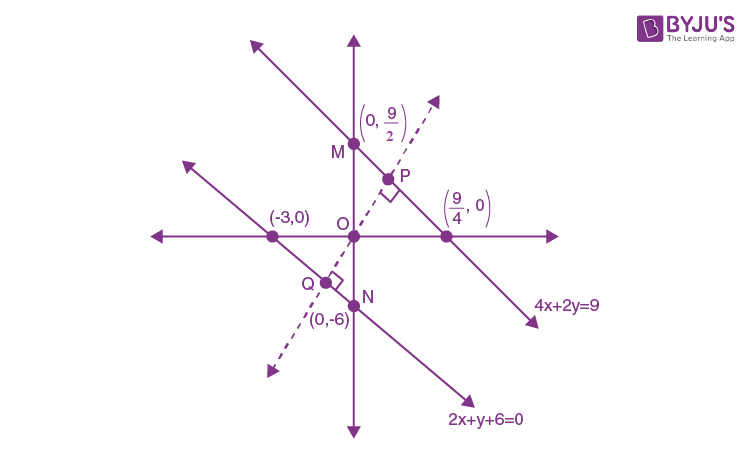ΔOPM ~ ΔOQN ⇒ OP/OQ = OM/ON

OP/OQ = 9/2/6

OP/OQ = 3/4

Question 37. Area in the first quadrant between the ellipse x2 + 2y2 – a2 and 2x2 + y2 – a2 is

1. a. a2/√2 tan-11/√2
2. b. 3a2/4 tan-11/2
3. c. 5a2/2 sin-11/2
4. d. 9πa2/2

Solution:

C1: x2 + 2y2 = a2

C2: 2x2 + y2 = a2

To find intersection point

P = (a/√3, a/√3)

Q = (a/√3, -a/√3)

R = (-a/√3, -a/√3)

S = (-a/√3, -a/√3)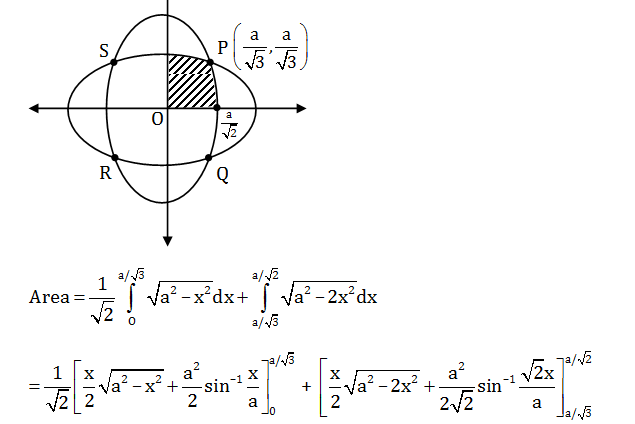= (a2/√2) tan-11/√2

Question 38. The equation of circle of radius √17 unit, with centre on the positive side of x-axis and through the point (0, 1) is

1. a. x2 + y2 – 8x – 1 = 0
2. b. x2 + y2 + 8x – 1 = 0
3. c. x2 + y2 – 9y + 1 = 0
4. d. 2x2 + 2y2 – 3x + 2y = 0

Solution:

Given that

Centre on positive side of x-axis = (a, 0) (a > 0)

So equation of circle: (x-a)2+ (y-0)2 = (√17)2

(x-a)2 + y2 = 17

As, it passes through (0, 1)

So (0 – a)2+(1)2 = 17

⇒ a2 + 1 = 17

⇒ a2 = 16

⇒ a = 4 {Since a > 0}

⇒ Equation of circle is

⇒ (x – 4)2 + y2 = 17

⇒ x2 – 8x + y2 + 16 = 17

⇒ x2 + y2 – 8x –1 = 0

Question 39. The length of the chord of the parabola y2 =4ax (a > 0) which passes through the vertex and makes an acute angle α with the axis of the parabola is

1. a. ±4a cot α cosec α
2. b. 4a cot α cosec α
3. c. -4a cot α cosec α
4. d. 4a cosec2 α

Solution: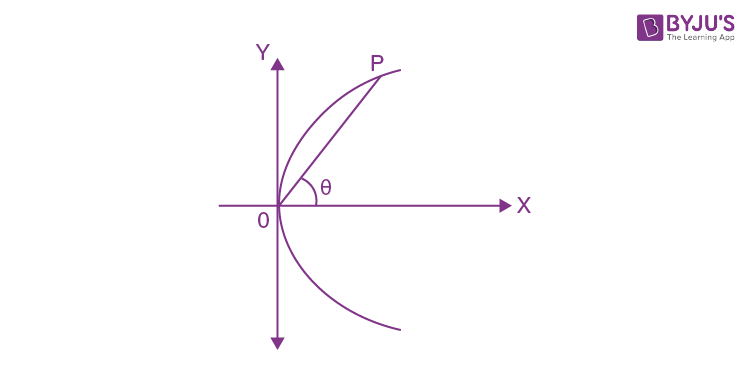Equation OP is: (y – 0) = tan θ (x –0)

y = x tan θ

Solving with y2 = 4ax, we get

⇒ (x tan θ)2 = 4ax

x2 tan2 θ = 4ax

x tan2 θ = 4a

x = 4a cot2 θ

Substituting, y = (4a cot2 θ) (tan θ)

y = 4a cot θ

Hence, P = (4a cot2 θ, 4a cot θ)

So, OP = √(0-4a cot2 θ)2+(0-4a cot θ)2

OP = 4a cot θ cosec θ

As 00 < θ < 900 so cot θ > 0, cosec θ > 0

Question 40. A double ordinate PQ of the hyperbola (x2/a2)-(y2/b2) = 1 is such that Δ OPQ is equilateral, O being the centre of the hyperbola. Then the eccentricity e satisfies the relation

1. a. 1 < e < 2/√3
2. b. e = 2/√3
3. c. e = √3/2
4. d. e > 2/√3

Solution: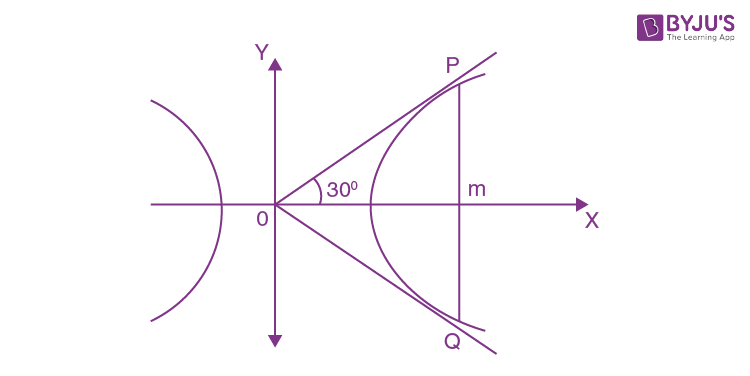Let P = (a sec θ, b tan θ) and Q = (a sec θ, – b tan θ)

In Δ OPM

tan 300 = b tan θ/a sec θ

1/ √3 = b sin θ/a

⇒ a/b = √3 sin θ

Eccentricity e2 = 1 + b2/a2

e2 = 1+ (1/3) sin2 θ

e2 > 1+ 1/3 {since max sin2 θ = 1}

e2 > 4/3

e > 2/ √3

Question 41. If B and B’ are the ends of the minor axis and S and S’ are foci of the ellipse (x2/25)+(y2/9) = 1, then the area of the rhombus SBS’ B’ will be

1. a. 12 sq. unit
2. b. 48 sq. unit
3. c. 24 sq. unit
4. d. 36 sq. unit

Solution:

(x2/25)+(y2/9) = 1

a2 = 25 ⇒ a = ± 5

b2 = 9 ⇒ b = ± 3

Eccentricity (e) = √(1-b2/a2)

= √(1-9/25)

= 4/5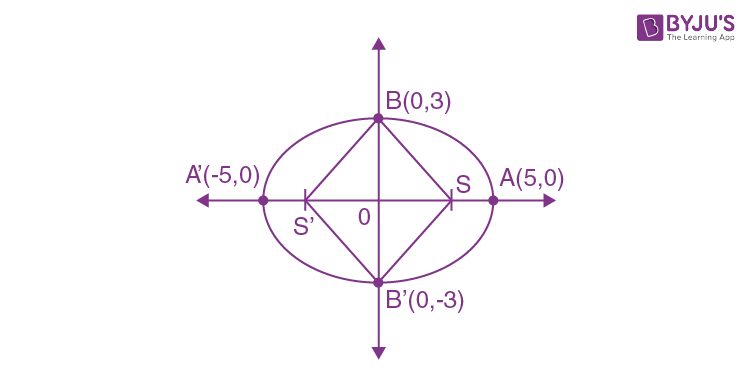Since S and S’ foci of the ellipse

S = (ae, 0) = (±5×4/5, 0) = (±4, 0)

Area of Rhombus = 4 × area of Δ BOS

= 4×(1/2)OS×OB

= 4×(1/2)4×3

= 24 sq. units

Question 42. The equation of the latus rectum of a parabola is x+y = 8 and the equation of the tangent at the vertex is x+y = 12. Then the length of the latus rectum is

1. a. 4√2 units
2. b. 2 √2 units
3. c. 8 units
4. d. 8√2 units

Solution:

Given that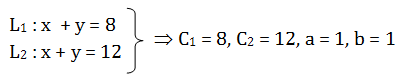The distance between latus rectum and the equation of tangent at vertex is ‘a’.

Here

$$a=\left | \frac{C_{1}-C_{2}}{\sqrt{a^{2}+b^{2}}} \right |$$

$$a=\left | \frac{8-12}{\sqrt{1+1}} \right |$$

a = 4/√2

a = 2√2

Hence, length of latus rectum is

LR = 4a

= 4(2√2)

= 8√2 Units

Question 43. The equation of the plane through the point (2, –1, –3) and parallel to the lines (x-1)/2= (y+2)/3 = z/-4 and x/2 = (y-1)/-3 = (z-2)/2 is

1. a. 8x + 14y + 13z + 37 = 0
2. b. 8x – 14y – 13z – 37 = 0
3. c. 8x – 14y – 13z + 37 = 0
4. d. 8x – 14y + 13z + 37 = 0

Solution:

Bonus

Question 44. The sine of the angle between the straight line (x-2)/3 = (y-3)/4 = (z-4)/5 and the plane

2x–2y + z = 5 is

1. a. 2√3/5
2. b. √2/10
3. c. 4/5√2
4. d. √5/6

Solution:

Given that

Line (x-2)/3 = (y-3)/4 = (z-4)/5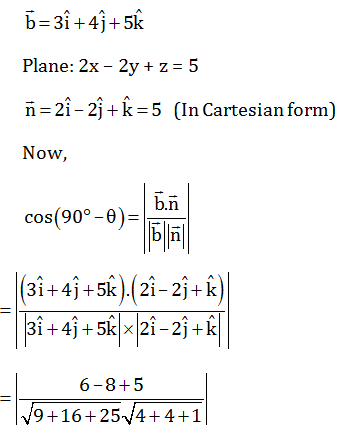=

$$\left | \frac{3}{5\sqrt{2}.3} \right |$$

= √2/10

Question 45. Let f(x) = sin x + cos ax be periodic function. Then

1. a. ‘a’ is real number
2. b. ‘a’ is any irrational number
3. c. ‘a’ is any rational number
4. d. a = 0

Solution:

f(x) = sinx + cos ax

Period of sinx + cos ax is LCM of 1 and a but LCM of rational multiple of same irrational is defined.

Question 46. The domain of f(x) = √((1/√x)-(√(x+1)) is

1. a. x> -1
2. b. (-1, ∞)\{0}
3. c. [0, (√5-1)/2]
4. d. [(1-√5)/2, 0)

Solution:

f(x) = √((1/√x)-( √(x+1))

x > 0, x + 1 ≥ 0 ⇒ x ≥ –1

and 1/√x-√(x+1) ≥ 0

⇒ (1/√x) ≥√(x+1)

Squaring both side

⇒ (1/x) ≥(x+1)

⇒ x2+x-1≤0

x = (-1±√(1-4(1)(-1)))/2(1)

= (-1±√5)/2

Therefore, x ∈(0, (√5-1)/2]

Question 47. Let y = f(x) = 2x2–3x + 2. The differential of y when x changes from 2 to 1.99 is

1. a. 0.01
2. b. 0.18
3. c. –0.05
4. d. 0.07

Solution:

Δ x = –0.01{Because, changes from 2 to 1.99}

f(x) = 2x2-3x + 2

Differentiate w.r.t. x

f’(x) = 4x – 3

Now,

Δ y = f’(x)Δ x

Δ y = f’(2)(–0.01)

Δ y = [4(2) – 3] (–0.01)

Δ y = –0.05

Question 48. If

$$\lim_{x\to\infty }\left [ \frac{1+cx}{1-cx} \right ]^{\frac{1}{x}}$$
= 4, then
$$\lim_{x\to\infty }\left [ \frac{1+2cx}{1-2cx} \right ]^{\frac{1}{x}}$$
is

1. a. 2
2. b. 4
3. c. 16
4. d. 64

Solution:

Given

$$\lim_{x\to\infty }\left [ \frac{1+cx}{1-cx} \right ]^{\frac{1}{x}}$$
= 4

Since 1 form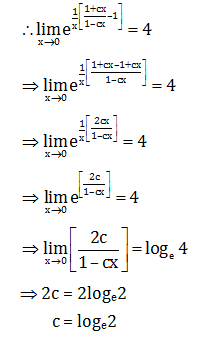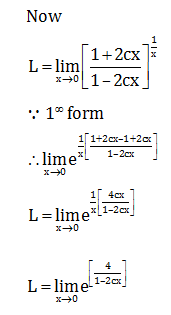L = e4c/1

L = e4c

= e4log 2

= 16

Question 49. Let f : R->R be twice continuously differentiable (or f” exists and is continuous) such that f(0) = f(1) = f’(0) = 0. Then

1. a. f”(c) = 0 for some c ∈ R
2. b. there is no point for which f” (x) = 0
3. c. at all points f”(x) > 0
4. d. at all points f”(x) < 0

Solution:

Consider f(x) on [0, 1]

f(x) be the twice continuously differentiable function.

Applying Rolle’s theorem on the interval [0, 1].

f’(a) = 0 for some a ∈ (0, 1)

Now, applying Rolle’s theorem to f’(x) on the interval [0, a]

f”(c) = 0 for some c ∈ (0, a)

Question 50. Let f(x) = x13+x11+x9+x7+x5+x3+x+12. Then

1. a. f(x) has 13 non-zero real roots
2. b. f(x) has exactly one real root
3. c. f(x) has exactly one pair of imaginary roots
4. d. f(x) has no real root

Solution:

Given

f(x) = x13 + x11 + x9 + x7 + x5 + x3 + x + 12

Differentiate w.r.t. x

f’(x) = 13x12 +11x10 + 9x8 + 7x6 + 5x4 + 3x2 + 1

f’(x) = ‘+’

f’(x) > 0

i.e. monotonically increasing for all x ∈ R

f(x) = 0 has exactly one real root

⇒f(x) intersect x-axis at only one point

Therefore, exactly one solution.

Question 51. Let z1 and z2 be two imaginary roots of z2 + pz +q = 0, where p and q are real. The points z1, z2 and origin form an equilateral triangle if

1. a. p2 > 3q
2. b. p2 < 3q
3. c. p2 = 3q
4. d. p2 = q

Solution:

Given that

z2 + pz + q = 0

since z1 and z2 are the roots of given equation.

Sum of root = z1 + z2 = –p

Product of roots = z1z2 = q

If z1, z2, z3 are the vertices of an equilateral triangle then z12+ z22+ z32 = z1z2+ z2z3+ z3z1

If z3 is origin then z12+ z22 = z1z2

⇒ z12+ z22 +2 z1z2 = z1z2+2 z1z2

⇒ (z1+z2)2 = 3z1z2

⇒ (–p)2 = 3q

p2 = 3q

Question 52. If the vectors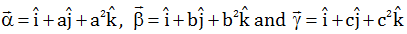are three non-coplanar vectors and

$$\begin{vmatrix} a & a^{2} &1+a^{3} \\ b & b^{2}& 1+b^{3}\\ c & c^{2} &1+c^{3} \end{vmatrix}$$
= 0, then the value of abc of

1. a. 1
2. b. 0
3. c. –1
4. d. 2

Solution:

Given

$$\begin{vmatrix} a & a^{2} &1+a^{3} \\ b & b^{2}& 1+b^{3}\\ c & c^{2} &1+c^{3} \end{vmatrix}$$
= 0

$$\begin{vmatrix} a & a^{2} &1 \\ b & b^{2}& 1\\ c & c^{2} &1 \end{vmatrix}+\begin{vmatrix} a & a^{2} &a^{3} \\ b & b^{2}& b^{3}\\ c & c^{2} &c^{3} \end{vmatrix}=0$$

$$\begin{vmatrix} a & a^{2} &1 \\ b & b^{2}& 1\\ c & c^{2} &1 \end{vmatrix}+abc\begin{vmatrix} 1 & a &a^{2} \\ 1 & b& b^{2}\\ 1 & c &c^{2} \end{vmatrix}=0$$

$$(1+abc)\begin{vmatrix} 1 & a &a^{2} \\ 1 & b& b^{2}\\ 1 & c &c^{2} \end{vmatrix}=0$$

$$\begin{vmatrix} 1 & a &a^{2} \\ 1 & b& b^{2}\\ 1 & c &c^{2} \end{vmatrix}$$
≠ 0 { a,b,c are three non coplanar vectors}

∴abc+1 = 0

abc = -1

Question 53. If the line y = x is a tangent to the parabola y = ax2 + bx + c at the point (1,1) and the curve passes through (–1, 0), then

1. a. a = b = –1, c = 3
2. b. a = b = 1/2 , c = 0
3. c. a = c = 1/4 , b = 1/2
4. d. a = 0, b = c = 1/2

Solution:

Given that

C1: y = ax2+bx+c

Differentiate w.r.t. x

dy/dx = 2ax+b.....(1)

Given

Line: y = x

dy/dx = 1 .....(2)

Since slopes are equal

∴ 2ax + b = 1

At (1, 1)

2a + b = 1.....(3)

Now, (1, 1) and (–1, 0) satisfies the curve

a + b + c = 1.....(4)

a– b + c = 0.....(5)

Equation (5) – equation (4)

⇒ a – b + c – a – b – c = 0 – 1

⇒ –2b = –1

b = 1/2

hence a = 1/4 {From equation (3)}

c = 1/4

Question 54. In an open interval, (0, π/2)

1. a. cos x + x sin x < 1
2. b. cos x + x sin x > 1
3. c. no specific order relation can be ascertained between cos x + x sin x and 1
4. d. cos x + x sin x > 1

Solution:

f(x) = cos x + x sin x – 1

Differentiate w.r.t. x

f’(x) = –sin x + sin x + x cos x > 0 ; x∈(0, π/2)

f(x) is increasing function

f(x) > f(0)

cos x+x sin x-1 > 0

cos x+x sin x > 1

Question 55. The area of the region {(x,y) : x2 + y2 ≤ 1 ≥ x + y} is

1. a. π2/2
2. b. π/4
3. c. (π/4)-(1/2)
4. d. π2/3

Solution:

Given that

C1: x2 + y2 = 1

C2: x + y = 1

To find intersection point

⇒ x2 +(1–x)2 = 1

x2 +1–2x + x2 = 1

2x2–2x = 0

x2–x = 0

x(x – 1) = 0

x = 0, 1

For x = 0

0+y = 1

So y = 1

A = (0,1)

For x = 1

1+y = 1

So y = 0

B = (1,0)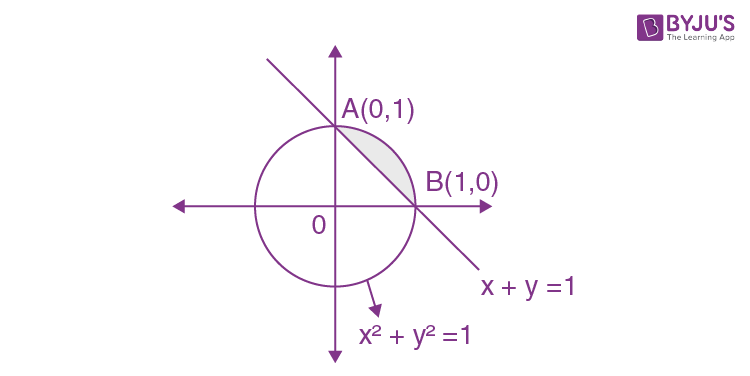Area = 1/4 (area of circle) – area of ΔAOB

= ¼( π)12-(1/2)1(1)

= (π/4)-(1/2) sq. units.

Question 56. If P(x) = ax2 +bx + c and Q(x) = – ax2 + dx + c, where ac ≠ 0 [a, b, c, d are all real], then P(x). Q(x) = 0 has

1. a. at least two real roots
2. b. two real roots
3. c. four real roots
4. d. no real root

Solution:

Given

P(x) = ax2 + bx + c

D1 = b2 – 4ac

Q(x) = –ax2 + dx + c

D2 = d2 + 4ac

⇒ D1 + D2

⇒ b2 – 4ac + d2 + 4ac

⇒ b2 + d2 > 0

At least two real roots.

Question 57. Let A = {x ∈R : –1 ≤x ≤1} & f : A -> A be a mapping defined by f(x) = x|x|. Then f is

1. a. Injective but not surjective
2. b. Surjective but not injective
3. c. Neither injective nor surjective
4. d. bijective

Solution:

f(x) = x |x|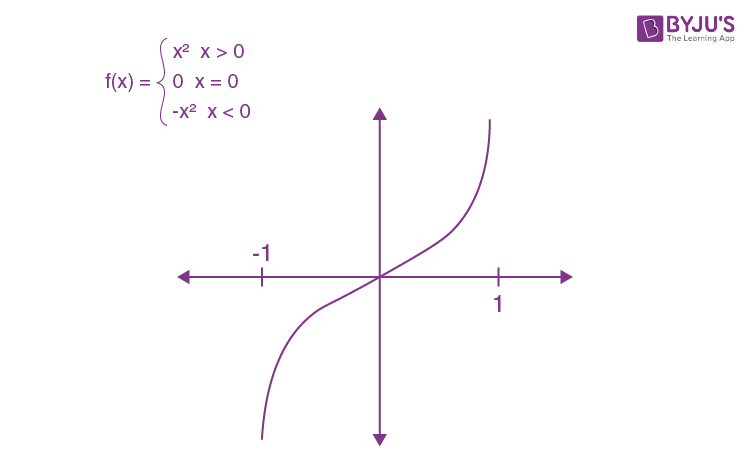∵ It is one-one and onto

∴ f(x) is bijective.

Question 58. Let f(x) = √(x2-3x+2) and g(x) = √x be two given functions. If S be the domain of f o g and T be the domain of g o f, then

1. a. S = T
2. b. S⋂T = φ
3. c. S⋂T is a singleton
4. d. S⋂T is an interval

Solution:

Given

f(x) = √(x2-3x+2)

g(x) = √x { since x > 0}

fog(x) = f[g(x)]

= f[√x ]

= √(x-3√x+2)

⇒ x –3√x+2 ≥ 0

x+2≥ 3√x

Squaring both side

⇒ (x + 2)2 ≥ (3√x )2

⇒ x2 +4x + 4≥9x

⇒ x2–5x + 4 ≥0

⇒ (x – 1) (x – 4) ≥0

Hence S = x ∈ (0,1] ⋃[4, ∞)

gof(x) = g[f(x)]

= g (√(x2-3x+2) )

= √(√(x2-3x+2) )

⇒ x2-3x+2 ≥0

⇒ (x – 1) (x – 2) ≥ 0

⇒ T = x ∈ (-∞, 1] ⋃[2, ∞)

Hence S⋂T = (0, 1] ⋃[4, ∞)

Question 59. Let ρ1 and ρ2 be two equivalence relations defined on a non-void set S. Then

1. a. both ρ1⋂ρ2 and ρ1 ⋃ ρ2 are equivalence relations
2. b. ρ1⋂ρ2 is equivalence relation but ρ1⋃ρ2 is not so
3. c. ρ1⋃ρ2 is equivalence relation but ρ1⋂ρ2 is not so
4. d. Neither ρ1⋂ρ2 nor ρ1⋃ρ2 is equivalence relation

Solution:

Given ρ1, ρ2 are equivalence relations on S.

⇒ ρ1, ρ2 are reflexive, symmetric and transitive.

Reflexive:

Let x ∈ S

⇒ (x, x) ∈ ρ1 and (x, x) ∈ ρ2

⇒ (x, x) ∈ ρ1⋂ρ2

⇒ ρ1⋂ρ2 is reflexive.

Symmetric:

Let (x,y) ∈ ρ1⋂ρ2

We have to show (y, x) ∈ ρ1⋂ρ2

(x, y) ∈ ρ1⋂ρ2

⇒ (x, y) ∈ ρ1 and (x, y) ∈ ρ2

⇒ (y, x) ∈ ρ1 and (y, x) ∈ ρ2

⇒ (y, x) ∈ ρ1⋂ρ2

⇒ ρ1⋂ρ2is symmetric

Transitive:

Let (x, y), (y, z) ∈ ρ1⋂ρ2

⇒ (x, y), (y, z) ∈ ρ1 and (x, y), (y, z) ∈ ρ2

⇒ (x, z) ∈ ρ1 and (x, z) ∈ ρ2

⇒ (x, z) ∈ ρ1 ⋂ρ2

⇒ ρ1 ⋂ρ2is transitive.

Therefore ρ1 ⋂ρ2is equivalence relation.

ρ1 ⋂ρ2 is always reflexive and symmetric but not transitive.

e.g. Let S = {1, 2, 3}

ρ1= {(1, 1), (2, 2) (3, 3), (1, 2), (2, 1)}

ρ2 = {(1, 1), (2, 2) (3, 3), (2, 3), (3, 2)}

ρ1, ρ2 is equivalence relation.

But ρ1⋃ρ2 is not transitive as (1, 2), (2, 3) ∈ ρ1 ⋂ρ2 but (1, 3) not belongs to ρ1⋃ρ2.

Question 60. Consider the curve (x2/a2)+(y2/b2) = 1. The portion of the tangent at any point of the curve intercepted between the point of contact and the directrix subtends at the corresponding focus an angle of :

1. a. π/4
2. b. π/3
3. c. π/2
4. d. π/6

Solution:

Ellipse: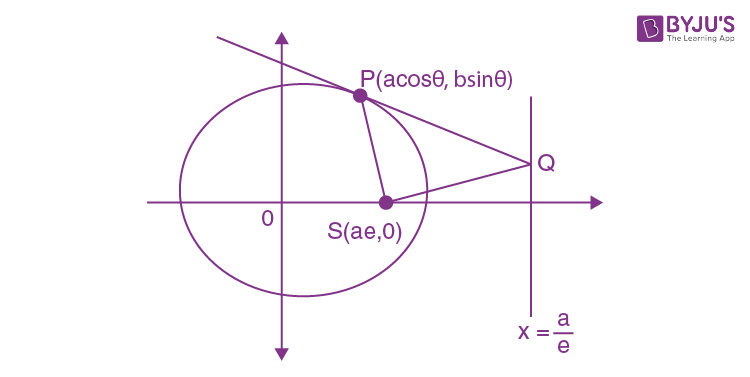Equation of tangent at any point P(a cos θ, b sin θ) on the ellipse is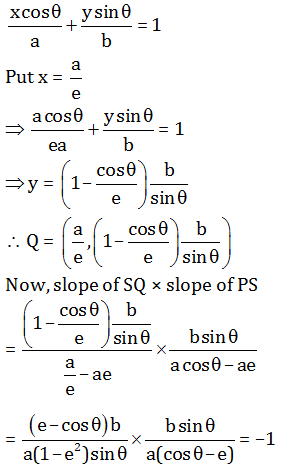So, the angle between the line PS and SQ is π/2.

Question 61. A line cuts x-axis at A(7,0) and the y-axis at B(0, –5). A variable line PQ is drawn perpendicular to AB cutting the x-axis at P(a, 0) and the y-axis at Q(0,b). If AQ and BP intersect at R, the locus of R is

1. a. x2 + y2 + 7x + 5y = 0
2. b. x2 + y2 + 7x – 5y = 0
3. c. x2 + y2 – 7x + 5y = 0
4. d. x2 + y2 – 7x – 5y = 0

Solution: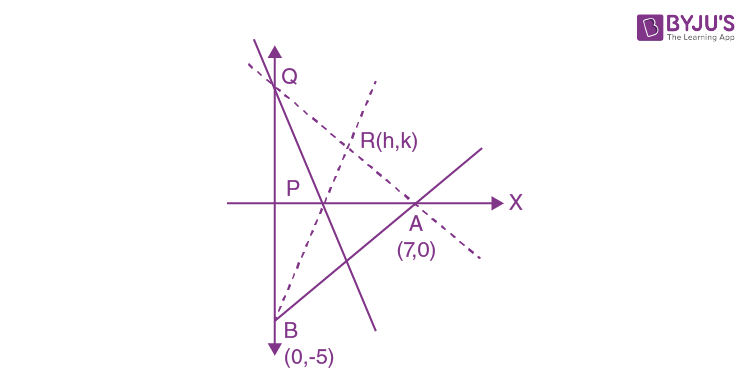P is orthocenter of ΔABQ

mBR × mAR = –1

⇒ (k+5)/(h-0) ×(k-0)/(h-7) = -1

⇒ k(k+5)/h(h-7)= –1

⇒k2+5k = –h(h–7)

⇒ k2+5k = –h2 + 7h

⇒ h2+ k2+5k -7h = 0

⇒ x2+y2+5y-7x = 0

Question 62. Let 0 < α < β < 1. Then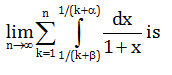1. a. loge β/α
2. b. loge (1+β)/(1+α)
3. c. loge (1+α)/(1+β)
4. d. ∞

Solution: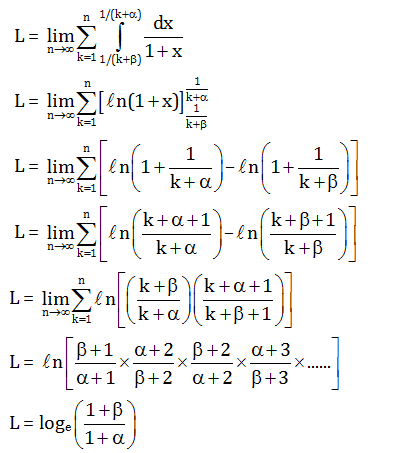Question 63.

$$\lim_{x\to 1}\left [ \frac{1}{ln\: x}-\frac{1}{x-1} \right ]$$

1. a. Does not exist
2. b. 1
3. c. 1/2
4. d. 0

Solution:

Let L =

$$\lim_{x\to 1}\left [ \frac{1}{ln\: x}-\frac{1}{x-1} \right ]$$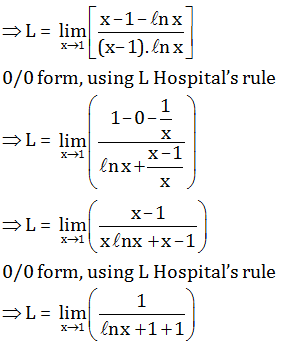L = (0+1)/2

= 1/2

Question 64. Let y = 1/(1+x+ln x), then

1. a. x (dy/dx)+y = x
2. b. x (dy/dx) = y(y ln x-1)
3. c. x2 (dy/dx) = y2+1-x2
4. d. x[dy/dx]2 = y-x

Solution:

y = 1/(1+x+ln x)

Dfferentiate w.r.t.x

dy/dx = [-1/(1+x+ln x)2](1+1/x)

dy/dx = -y2(x+1)/x

x dy/dx = -y2(x+1)

⇒ x dy/dx = -y2(1/y - ln x)

⇒ x dy/dx = -y+y2ln x

⇒ x dy/dx = y(y ln x-1)

Question 65. Consider the curve y = be–x/a where a and b are non-zero real numbers. Then

1. a. (x/a)+(y/b) = 1 is tangent to the curve at (0,0)
2. b. (x/a)+(y/b) = 1 is tangent to the curve where the curve crosses the axis of y
3. c. (x/a)+(y/b) = 1 is tangent to the curve at (a, 0)
4. d. (x/a)+(y/b) = 1 is tangent to the curve at (2a, 0)

Solution:

Given

y = be–x/a

Differentiate w.r.t. x

dy/dx = (-b/a) e–x/a

Tangent: (x/a)+(y/b) = 1

Slope of the tangent (1/a)+(1/b)dy/dx = 0

(dy/dx) = -b/a

So, (x/a)+(y/b) = 1 is tangent to the curve at the point where x = 0 ⇒ y = b

So, (x/a)+(y/b) = 1 is a tangent to the curve at the point (0, b)

Question 66. The area of the figure bounded by the parabola x = –2y2, x = 1–3y2 is

1. a. 1/3 square unit
2. b. 4/3 square unit
3. c. 1 square unit
4. d. 2 square unit

Solution:

C1: x = –2y2

C2: x = 1–3y2

To find intersecting point

⇒ –2y2 = 1–3y2

⇒ y2 = 1

y = ± 1

So, x = –2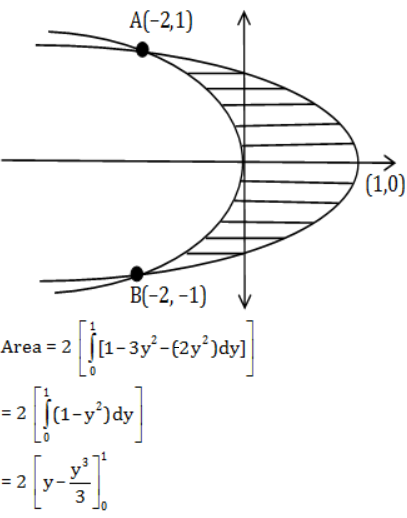= 2(1-1/3)

= 4/3 square units.

Question 67. A particle is projected vertically upwards. If it has to stay above the ground for 12 seconds, then

1. a. velocity of projection is 192 ft/sec
2. b. greatest height attained is 600 ft
3. c. velocity of projection is 196 ft/sec
4. d. greatest height attained is 576 ft

Solution:

If the initial velocity is u ft/sec then time taken for the entire motion is: t = 2u/g

g = 32 ft/sec2 and t = 12 second

t = 2u/g

⇒ 12 = 2u/32

⇒ u = 192

Greatest height attained

H = u2/2g

⇒ H = (192)2/2(32)

⇒ H = 576

Question 68. The equation

$$x^{(log_{3}x)^{2}-\frac{9}{2}log_{3}x+5}=3\sqrt{3}$$

1. a. at least one real root
2. b. exactly one real root
3. c. exactly one irrational root
4. d. complex roots

Solution:

$$x^{(log_{3}x)^{2}-\frac{9}{2}log_{3}x+5}=3\sqrt{3}$$

Let log3x = t

x = 3t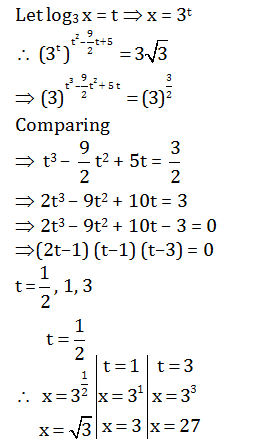Hence x = √3 , 3, 27 are the roots of the given equation.

Question 69. In a certain test, there are n questions. In this test 2n–I students gave wrong answers to at least i questions, where i = 1,2…… , n. If the total number of wrong answer given is 2047, then n is equal to

1. a. 10
2. b. 11
3. c. 12
4. d. 13

Solution:

The number of students answering exactly i

(1 ≤ I ≤ n – 1) questions wrongly is 2n–i –2n–i–1

Thus, the total number of wrong answer is

1(2n–1–2n–2) + 2(2n–2–2n–3) + ……+ (n–1) (21–20) + n(20) +……

= 2n–1 + 2n–2 +2n–3 + ….. + 20 = 2n –1

Thus, 2n–1 = 2047

⇒ 2n = 2048

⇒ 2n = 211

⇒ n = 11

Question 70. A and B are independent events. The probability that both A and B occur is 1/20 and the probability that neither of them occurs is 3/5. The probability of occurrence of A is

1. a. 1/2
2. b. 1/10
3. c. 1/4
4. d. 1/5

Solution:

1. Answer: (c, d)

P(A’⋂B’) = 3/5

1-P(A⋃B) = 3/5

P(A ⋃ B) = 2/5

P(A) + P(B) – P(A) P(B) = 2/5

P(A) + P(B) = 2/5 + 1/20 {since P(A)P(B) = 1/20 ⇒ P(B) = 1/20P(A) }

P(A) + P(B) = 9/20

P(A) + 1/20P(A) = 9/20

20[P(A)]2 + 1= 9 P(A)

20[P(A)]2 – 9P(A) +1 = 0

(4P(A)–1) (5 P(A)–1) = 0

P(A) = ¼, 1/5

Question 71. The equation of the straight line passing through the point (4, 3) and making intercepts on the co-ordinate axes whose sum is –1 is

1. a. x/2 -y/3 = 1
2. b. x/-2+y/1 = 1
3. c. -x/3+y/1 = 1
4. d. x/1-y/2 = 1

Solution:

Equation of a line making an x-intercept = ‘a’ units and y – intercept = ‘b’ units is given by

x/a + y/b = 1 …….(1)

Also, a + b = –1 …..(2)

And equation (1) passes through point (4, 3)

So 4/a+3/b = 1 …..(3)

From (2), b = –a–1 …..(4)

Substituting eq.(4) in eq. (3) we get

4/a+3/-a-1 = 1

4/a-3/a+1 = 1

4a+4-3a = a(a+1)

a + 4 = a2 + a

⇒ a = ± 2

So a = 2, a = –2

b = –3, b = 1

So the probable equation will be

x/2 -y/3 = 1 and -x/2+y/1 = 1

Question 72. Let f(x) = x sin x – (1–cos x). The smallest positive integer k such that

$$\lim_{x\to0}\frac{f(x)}{x^{k}}\neq 0$$
is

1. a. 4
2. b. 3
3. c. 2
4. d. 1

Solution:

Given f(x) = x sin x-(1–cos x).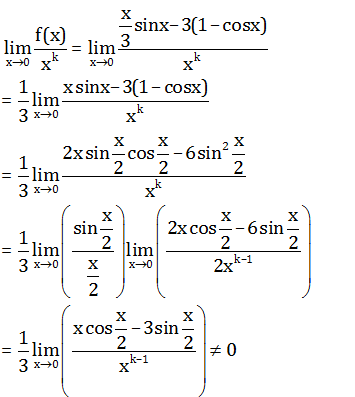⇒ k – 1 = 1

⇒ k = 2

Question 73. Consider a tangent to the ellipse x2/2+y2/1 = 1 at any point. The locus of the midpoint of the portion intercepted between the axes is

1. a. x2/2+y2/4 = 1
2. b. x2/4+y2/2 = 1
3. c. 1/3x2+1/4y2 = 1
4. d. 1/2x2+1/4y2 = 1

Solution: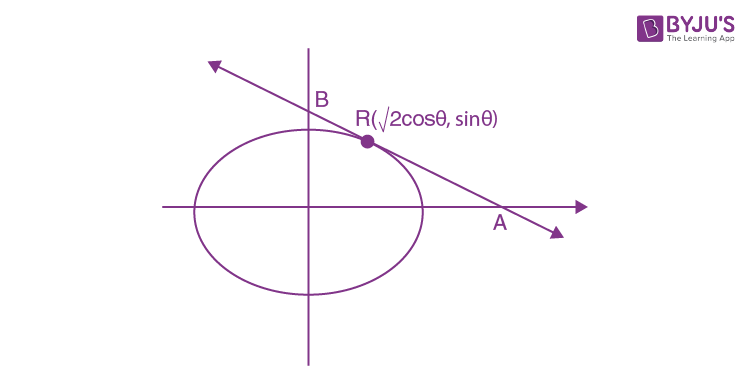Tangent at R (√2sin θ, cos θ)

(x√2cos θ )/2 +(y sin θ )/1 = 1

A = (√2/cos θ,0), B = (0, 1/sin θ)

Let P(h, k) be the locus of the mid point

So (h, k) = (√2/2 cos θ,1/2 sin θ)

cos θ = 1/√2h, sin θ = 1/2k

⇒ sin2 θ + cos2 θ = 1

⇒ 1/2h2 +1/4k2 = 1

So locus of (h, k) is 1/2x2+1/4y2 = 1

Question 74. Tangent is drawn at any point P(x, y) on a curve, which passes through (1, 1). The tangent cuts X-axis and Y-axis at A and B respectively. If AP : BP = 3 : 1, then

1. a. the differential equation of the curve is 3x(dy/dx)+ y = 0
2. b. the differential equation of the curve is 3x(dy/dx)– y = 0
3. c. the curve passes through (1/8, 2)
4. d. the normal at (1, 1) is x+3y = 4

Solution: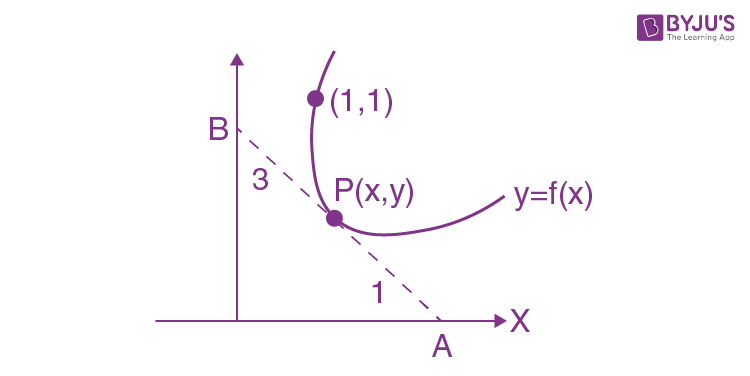Since, BP: AP = 3 : 1, then equation of tangent is

Y – y = f’x (X–x)

The intercept on the coordinate axes are

A = (x-y/f’(x) -0) and B(0, y-x f’(x))

Since, P is internally intercepts a line AB

So x = (3(x-y/f’(x))+1 ×0)/(3+1)

dy/dx = y/-3x

dy/dy = (-1/3x)dx

On integrating both sides, we get xy3 = c

Since, curves passes through (1, 1), then c = 1

So xy3 = 1

At x = 1/8

y = 2

Question 75. Let y = x2/(x+1)2(x+2). Then d2y/dx2 is

1. a. 2[3/(x+1)4 -3/(x+1)3+4/(x+2)2]
2. b. 3[2/(x+1)3 +4/(x+1)2+5/(x+2)3]
3. c. [6/(x+1)3 -4/(x+1)2+3/(x+1)2]
4. d. [7/(x+1)3 -3/(x+1)2+2/(x+1)3]

Solution:

y = x2/(x+1)2(x+2)

Using partial fraction concept

y = A/(x+1)+B/(x+1)2+C/(x+2)

⇒ x2/(x+1)2(x+2) = [A(x+1)(x+2)+B(x+2)+C(x+1)2 ]/(x+1)2(x+2)

⇒ x2/(x+1)2(x+2) = [x2(A+C)+x(3A+B+2C)+(2A+2B+C)]/(x+1)2(x+2)

Comparing

A = –3, B = 1, C = 4

Hence y = -3/(x+1)+1/(x+1)2+4/(x+2)

Differentiating w.r.t.x

dy/dx = 3/(x+1)2-2/(x+1)3-4/(x+2)2

Again differentiating w.r.t.x

d2y/dx2 = -6/(x+1)3+6/(x+1)4+8/(x+2)3

⇒ d2y/dx2 = 2[-3/(x+1)3+3/(x+1)4+4/(x+2)3]

### WBJEE 2020 Maths Question Paper with Solutions